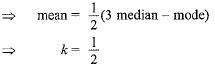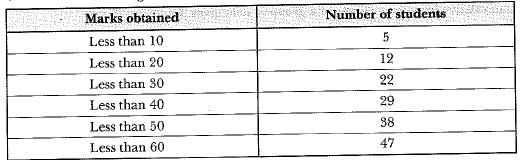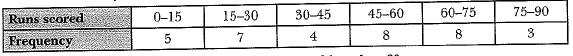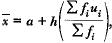Courses

# MCQ : Statistics - 2

## 10 Questions MCQ Test Mathematics (Maths) Class 10 | MCQ : Statistics - 2

Description
This mock test of MCQ : Statistics - 2 for Class 10 helps you for every Class 10 entrance exam. This contains 10 Multiple Choice Questions for Class 10 MCQ : Statistics - 2 (mcq) to study with solutions a complete question bank. The solved questions answers in this MCQ : Statistics - 2 quiz give you a good mix of easy questions and tough questions. Class 10 students definitely take this MCQ : Statistics - 2 exercise for a better result in the exam. You can find other MCQ : Statistics - 2 extra questions, long questions & short questions for Class 10 on EduRev as well by searching above.
QUESTION: 1

Solution:
QUESTION: 2

### Mode and mean of a data are 12k and 15k. Median of the data is

Solution:

∵ Mode = 3 median - 2 mean
⇒ 12k = 3 median - 2 x 15k
⇒ 42k = 3 median
⇒ Median = 14k.

QUESTION: 3

### The class mark of the class 15.5 - 20.5

Solution:
QUESTION: 4

If mean = (3 median - mode). k, then the value of k is

Solution:

∵ Mode = 3 median - 2 mean
⇒ 2 mean = 3 m edian - modeQUESTION: 5

Consider the following distribution:The frequency of the class 50 - 60 is

Solution:
QUESTION: 6

The abscissa of the point of intersection of both types (less than & more than) of cumulative frequency curves help in finding

Solution:
QUESTION: 7

The runs scored by a batsman in 35 different matches are given below:The number of matches in which the batsman scored less than 60 runs are

Solution:
QUESTION: 8

The class marks of the class 18-22 is

Solution:
QUESTION: 9

In the formulafor finding the mean of a grouped frequency distribution, ui =

Solution:
QUESTION: 10

The arithmetic mean of 1, 2, 3 ...... n is

Solution: